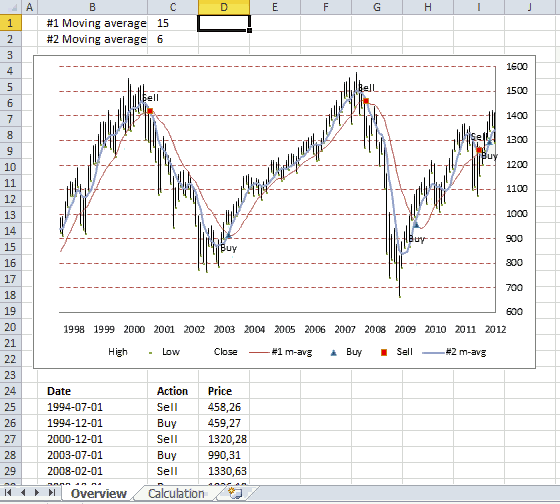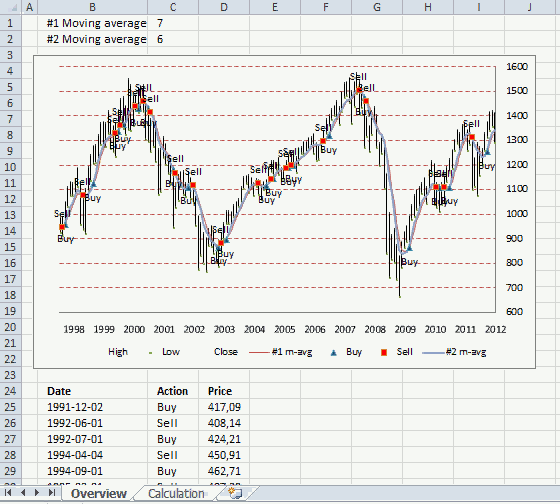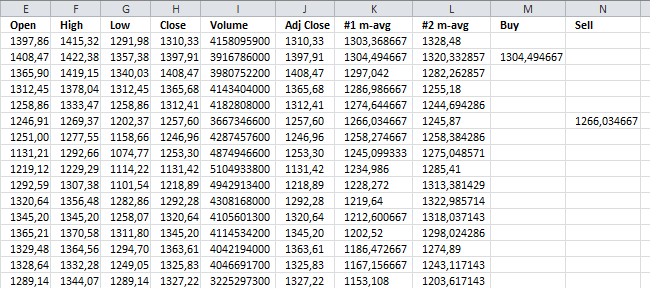Author: Oscar Cronquist Article last updated on September 06, 2019This article demonstrates how to display buy and sell signals on an Excel chart based on two moving averages, the workbook lets you change how these moving averages are calculated. There is a download link below.Above is an animated gif demonstrating a stock chart of S&P 500 with monthly prices. Two moving averages #1, #2, buy and sell points are plotted in this chart.

When the moving averages intersects a buy or sell point is created. If you use the value 7 in cell C1, moving average #1 uses the average of 7 months.

As you can see using a 15 month average (#1) returns better and fewer buy and sell points.

### Extracting buy and sell points

Not only is the chart refreshed as you type new values in cell C1 and C2, the data below the chart is also instantly refreshed.

Array formula in cell B25:

=IFERROR(INDEX(Calculation!\$D\$2:\$D\$247, LARGE(IF((IFERROR(Calculation!\$M\$2:\$M\$247, "")<>"")+(IFERROR(Calculation!\$N\$2:\$N\$247, "")<>""), MATCH(ROW(Calculation!\$M\$2:\$M\$247), ROW(Calculation!\$M\$2:\$M\$247)), ""), ROW(A1))), "")

Array formula in cell C25:

=IFERROR(IF(INDEX(Calculation!\$M\$2:\$M\$300, MATCH(B25, Calculation!\$D\$2:\$D\$300, 0))="", "Sell", "Buy"), "")

Array formula in cell D25:

=IFERROR(INDEX(Calculation!\$H\$2:\$H\$300, MATCH(B25, Calculation!\$D\$2:\$D\$300, 0)), "")

How to create an array formula

1. Copy array formula above
2. Select cell B25
3. Paste formula to cell
4. Make sure you see the prompt in the cell, double click on the cell if you don't.
5. Press and hold Ctrl+ Shift simultaneously
6. Press Enter once
7. Release all keys

How to copy array formula

1. Select cell B25
2. Copy cell (not formula in formula bar)
3. Select cell range B26:B50
4. Paste

### Explaining formula in cell B25

#### Step 1 - Remove errors

The IFERROR function removes errors and returns an array containing values or nothing, errors are filtered out.

IFERROR(Calculation!\$M\$2:\$M\$247, "")

#### Step 2 - Check if cell is empty

The less than and greater than sign combined means "not equal to", in this case the logical operators make sure that the value is not equal to nothing. It returns boolean values TRUE or FALSE.

IFERROR(Calculation!\$M\$2:\$M\$247, "")<>""

#### Step 3 - Check column N as well

Actions described in step 1 and 2 are also applied to column N.

(IFERROR(Calculation!\$N\$2:\$N\$247, "")<>"")

#### Step 4 - If cells are not empty return the corresponding relative row number

The + sign between the logical expressions performs an OR calculation meaning if the cell in column M is not equal to nothing OR if the cell in column N is not equal to nothing then return TRUE, both logical expressions must return FALSE in order to return a FALSE.

(IFERROR(Calculation!\$M\$2:\$M\$247, "")<>"")+(IFERROR(Calculation!\$N\$2:\$N\$247, "")<>"")

The IF function then returns the second argument if the logical expression returns TRUE.

IF((IFERROR(Calculation!\$M\$2:\$M\$247, "")<>"")+(IFERROR(Calculation!\$N\$2:\$N\$247, "")<>""), MATCH(ROW(Calculation!\$M\$2:\$M\$247), ROW(Calculation!\$M\$2:\$M\$247)), "")

The second argument is the corresponding row number and the third argument is nothing.

MATCH(ROW(Calculation!\$M\$2:\$M\$247), ROW(Calculation!\$M\$2:\$M\$247)

#### Step 5 - Extract the k-th largest row number

The LARGE function extracts the k-th largest value in the array. LARGE( array, k)

LARGE(IF((IFERROR(Calculation!\$M\$2:\$M\$247, "")<>"")+(IFERROR(Calculation!\$N\$2:\$N\$247, "")<>""), MATCH(ROW(Calculation!\$M\$2:\$M\$247), ROW(Calculation!\$M\$2:\$M\$247)), ""), ROW(A1))

Argument k is a relative cell reference that changes when the cell is copied, this makes it possible to extract different values.

#### Step 6 - Return value from column D based on row number

The INDEX function returns a value from a cell range based on a row and column number.

INDEX(Calculation!\$D\$2:\$D\$247, LARGE(IF((IFERROR(Calculation!\$M\$2:\$M\$247, "")<>"")+(IFERROR(Calculation!\$N\$2:\$N\$247, "")<>""), MATCH(ROW(Calculation!\$M\$2:\$M\$247), ROW(Calculation!\$M\$2:\$M\$247)), ""), ROW(A1)))

### Calculating buy and sell points

Sheet: CalculationArray formula in cell K2:

=AVERAGE(OFFSET(H2, 0, 0, Overview!\$C\$1))

Array formula in cell L2:

=AVERAGE(OFFSET(H2, 0, 0, Overview!\$C\$2))

Formula in cell M2:

=IF((L3<K3)*(L2>K2), K2, "")

Formula in cell N2:

=IF((L3>K3)*(L2<K2), K2, "")

Copy cells and paste down as far as needed.

The calculation sheet imports historical prices using this user defined function:
Excel udf: Import historical stock prices from yahoo – added features

### Further reading

If you want to know how to plot buy and sell points in an excel stock chart, read this post:
Add buy and sell points to a stock chart

### Download Excel fileexcel-stock-chart-two-moving-averagesv2.xlsm